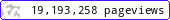•••••MadAsMaths.com :: I.Y.G.B. Practice Papers :: Core Practice Papers 2005 Spec :: C2 Practice Papers

I.Y.G.B. C2 PRACTICE PAPERS

These papers have been written recently and follow the Pearson/Edexcel Syllabus introduced in 2005. The students that have used these papers feel that they are slightly harder than the actual examination papers.

• Papers A to R have standard difficulty with later papers usually more difficult.

• Papers S and T are extremely hard.

• Papers U to Z are hard.

All papers have model solutions and most have guide marking schemes.

The difficulty rating (the second number indicated at the front of each paper) is a very rough UMS converter, so long as the total marks in the paper are 45 or more.

UMS = TOTAL MARKS multiplied by the DIFFICULTY RATING
e.g. total marks = 55
difficulty rating = 1.4033
U.M.S = 77 (approx).

 c2_a.pdfc2_a_marks.pdfc2_a_solutions.pdfc2_b.pdfc2_b_marks.pdfc2_b_solutions.pdfc2_c.pdfc2_c_marks.pdfc2_c_solutions.pdfc2_d.pdfc2_d_marks.pdfc2_d_solutions.pdfc2_e.pdfc2_e_marks.pdfc2_e_solutions.pdfc2_f.pdfc2_f_marks.pdfc2_f_solutions.pdfc2_g.pdfc2_g_marks.pdfc2_g_solutions.pdfc2_h.pdfc2_h_marks.pdfc2_h_solutions.pdfc2_i.pdfc2_i_marks.pdfc2_i_solutions.pdfc2_j.pdfc2_j_marks.pdfc2_j_solutions.pdfc2_k.pdfc2_k_marks.pdfc2_k_solutions.pdfc2_l.pdfc2_l_marks.pdfc2_l_solutions.pdfc2_m.pdfc2_m_marks.pdfc2_m_solutions.pdfc2_n.pdfc2_n_marks.pdfc2_n_solutions.pdfc2_o.pdfc2_o_marks.pdfc2_o_solutions.pdfc2_p.pdfc2_p_marks.pdfc2_p_solutions.pdfc2_q.pdfc2_q_marks.pdfc2_q_solutions.pdfc2_r.pdfc2_r_marks.pdfc2_r_solutions.pdfc2_s.pdfc2_s_marks.pdfc2_s_solutions.pdfc2_t.pdfc2_t_marks.pdfc2_t_solutions.pdfc2_u.pdfc2_u_marks.pdfc2_u_solutions.pdfc2_v.pdfc2_v_marks.pdfc2_v_solutions.pdfc2_w.pdfc2_w_marks.pdfc2_w_solutions.pdfc2_x.pdfc2_x_marks.pdfc2_x_solutions.pdfc2_y.pdfc2_y_marks.pdfc2_y_solutions.pdfc2_z.pdfc2_z_marks.pdfc2_z_solutions.pdf